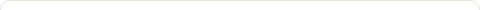# WBM1991: JAMES TOBIN LIQUIDITY PREFERENCE

Posted on: May 24, 2020

WBM POSTS ARE MY LOST WORKS FOUND IN THE WAY BACK MACHINE

### Tobin’s Liquidity Preference

Tobin, James: Liquidity Preference As Behavior Toward Risk, Review of Economic Studies, Vol. 25 1958 , pp. 65-86.

1. The Tobin 1958 paper is a landmark in the annals of Finance. A truly great paper does not only provide new evidence and interpretation but also gives new insight. Economist James Tobin brings together two great works – the liquidity preference theory of John Maynard Keynes and the portfolio theory of Harry Markowitz and shows that they are complementary and can be considered to be two manifestations of the same underlying principle of economics.
2. Keynes had postulated that in addition to cash needed to carry out transactions, EUs (Economic Units) also hold cash because of a speculative motive, that is, in case interest rates rise next year. He therefore concluded that the demand for money by the aggregate economy will rise and fall with the prevailing interest rate. When interest rates are high, EUs would be less inclined to think that they will rise any higher and will therefore invest in bonds and give up money. Conversely, when interest rates are low, the EUs would hold more cash and wait for higher rates on bonds.
3. So, if one plotted the demand for money against interest rate one would get the now well known textbook curve that slopes gently downward from all the money held at zero interest on bonds and asymptotically approaches zero money held at infinite interest. This description of liquidity preference has been severely criticized by Leontief and Miller.
4. Tobin, inspired by Markowitz, saw Keynes liquidity preference curve in terms of portfolio theory, i.e., the maximization of utility in mean-variance space. Tobin’s theory satisfied Leontiefs critique and brought liquidity preference into harmony with the mainstream of economic thought of his time. He proposes the following lucid and enlightening explanation of the downward sloping money curve.
5. Each EU holds a portfolio of two assets, \$(1-B) in money and \$B in bonds (note 2). B is less than unity and represents the value weighted percentage of the portfolio held in bonds. Money pays no interest and holds its value with certainty (note 3). Bonds sell today for \$1 and will pay a perpetuity of \$Ro. (i.e., Ro% interest rate).
6. However, next year, the prevailing interest rate may change to R1. At that time, a bond which pays \$Ro will sell for \$(Ro/R1) and the capital gain will be G=1-Ro/R1 since the EU paid \$1 for it. Thus the EUs net gain from holding a bond is interest + capital gain = Ro + G = Ro + 1 – Ro/R1. The assumption that g is normally distributed about an expected value of zero with a standard deviation of Sg leads to the following equations.
7. (a) Dollar value of net gains from holding the portfolio for 1 year: R = B*(Ro + G). (b) The expected value of R is: E(R) = E(B*Ro) + E(G) but E(G) = 0 therefore E(R) = B*Ro. The variance of R can be computed as var(R) = E{[R – E(R)]2} = E{[B*Ro + B*G – B*Ro]2} = E{B2G2}. Since G is normally distributed about zero (i.e., E(G) = 0), G2 = Sg2 and the standard deviation of R is given by: Sigma = sqrt(var(R)) = B*Sg. Rearranging B = Sr/Sg.
8. Now we can substitute for B in the equation for expected value. E(R) = B*Ro = Sr * (Ro/Sg) or E(R)/Sr = Ro/Sg = the market price of risk. This ratio which establishes the market price of risk in the aggregate economy is analogous to the security market line and is called the opportunity locus (OL) by Tobin.
9. Each EU in the economy is identified in the same mean-variance space by his own set of indifference curves (whose shape is defined by his peculiar attitude toward risk) and maximizes his mean-variance utility by holding a portfolio of money and bonds at a point in this space where his indifference curve is tangential to the OL. The point determines the fraction of his liquid wealth he holds in money and that which he holds in bonds.
10. With returns on the y-axis and risk on the x-axis, the OL gets steeper as interest rate rises. It is easy to see graphically, (and possible to show analytically as Tobin has done for all brave souls that have waded through section 3.3) that for riskophobes a steeper OL means that a larger fraction of the portfolio will be held in bonds easing the demand for cash. This is consistent with Keynes liquidity preference theory (increased interest rate leads to lower demand for money). QED.
11. Tobin wrestles with the fact that the relationship is reversed for riskophiles but offers no answers. One interpretation I can offer is that since empirical data show that the demand for money is indeed downward sloping, it must mean one of two things; either Tobins simple model of risk free money and perpetual bonds is too simplistic to yield results that can be compared directly with empirical data, or the implicit assumption of invariant prices (i.e. risk free cash) is not justified by the data. Of course, it could also mean that the empirical data suggests that the population at large is risk averse.

### 1 Response to "WBM1991: JAMES TOBIN LIQUIDITY PREFERENCE"Reblogged this on uwerolandgross.• Bree Bites Food: Loved reaading this thanks
• Laurence Hunt: Oh yeah, I reposted on Twitter.
• Laurence Hunt: I take global warming as a serious issue, though I favour private (investment-based) solutions to anticapitalism, government expansion and tax increas